Tuesday 31st March 2020

CBSE Guess > Papers > Question Papers > Class XII > 2006 > Chemistry > Delhi Set - I

Chemistry — 2006 (Set I — Delhi)

Q. 1. A cubic solid is made of two elements X and Y. Atoms V are at the corners of the cube and X at the body centre. What is the formula of the compound? (1)

Q. 2. Two liquids A and B boil at 145°C and 190°C respectively. Which of them has a higher vapour pressure at 80°C? (1)

Q. 3. For the reaction A → B, the rate of reaction becomes twenty seven times when the concentration of A is increased three times. What is the order of the reaction? (1)

Q. 4. Write the IUPAC name of: CH3.CH (Br) CH2CONHCH3. (1)

Q. 5. Give a chemical test to distinguish between aniline and N-methyl aniline. (1)

Q. 6.

1. Draw the structure of XeF2 molecule. (1)
2. Write the outer electronic configuration of Cr atom (Z = 24). (1)

Q. 7. What is meant by entropy driven reaction? How can a reaction with positive changes of enthalpy and entropy be made entropy driven? (2)

Q. 8. Write balanced chemical equations for the following reactions: (2)

1. Ca3P2 + H2O →
2. XeF6 + 3 H2O →

Q. 9. Write chemical equations 1 the reactions involved in the manufacture of potassium permanganate from pyrolusite ore (2)

Q. 10. What are enantiomers? Draw the structures of the possible enantiomers of 3-methyl pent-1-ene. (2)

Q. 11. Write the reactions and the conditions involved in the conversion of:

1. Propene to 1-Propanol (1)
2. Phenol to Salicyclic acid (1)

Q. 12. Write the structures of monomers used in the preparation of: (2)

1. Teflon
2. PMMA

Or

1. How does vulcanization change the character of natural rubber? (1)
2. Why are the numbers 66 and 6 put in the names of nylon-66 and nylon-6? (1)

Q. 13. State Heisenberg’s uncertainty principle. An electron has a velocity of 50 ms--1 accurate up to 99.99%. Calculate the uncertainty in locating its position? (Mass of electron = 9.1 x 10 --31 kg, h = 6.6 x 10 —34 J.s.) (1, 2)

Q. 14. An element has a body centered cubic structure with a cell .edge of 288 rm. The density of the element is 7.2 gcm 3 Calculate the number of atoms present in 208 g of the element. (3)

Q. 15.

1. Why is the vapour pressure of a solution of glucose in water lower than that of water? (1)
2. A 6.90 M solution of KOH in water contains 30% by mass of KOH. Calculate the density of the KOH solution?
[Molar mass of KOH = 56 g mol –1] (2)

Q. 16. Answer the following in brief:

1. Which of the two isomers of butane is more stable at 25°C and why?
Given [n-butane (∆ f H 0 = - 120 kJ mol --1) and isobutane (∆ f H ° =—130 kJ mol--1)]
2. For the change H20 (l) →H2O (g), predict the sign of ∆S. (1)
3. For the reaction N 2(g) + 3H2 (g) → 2NH3 (g), predict whether work is done by the system or on the system and why? (1)

Q. 17. The rate of a particular reaction triples when temperature changes from 50°C to 100°C. Calculate the activation energy of the reaction.
[log 3 = 0.4771; R = 8.314 JK --1 mol–I] (3)

Q. 18.

1. How can a colloidal solution and true solution of the same colour be distinguished from each other? (1)
2. List four applications of adsorption. (2)

Or

Explain the following observations:

1. Lyophilic colloid is more stable than Lyophobic colloid. (1)
2. Coagulation takes place when sodium chloride solution is added to a colloidal solution of ferric hydroxide. (1)
3. Sky appears blue in colour. (1)

Q. 19. Name the chief ore of silver. Describe with chemical equations the extraction of silver from this ore. (3)

Q. 20.

1. Using valence bond theory predict the geometry and magnetic behaviour of [Cr (NH3)6]3+ ion [Cr=24]. (2)
2. Write IUPAC name of:
[Pt (NH 3)2Cl2]

Q. 21.

1. The isotope of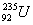decays in 14 steps, by loss of 8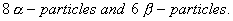What are mass number and atomic number of the end product? (1)
2. Write the equation for the complete reaction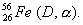(1)
3. How is the nuclear binding energy related to the stability of the nucleus? (1)

Q. 22.

1. Describe the following giving suitable examples: (2)
1. Cannizzaro reaction
2. Aldol condensation
2. Give a chemical test to distinguish between ethanal and propanal. (1)

Q. 23. Account for the following:

1. Electrophillic substitution in case of aromatic amines takes place more readily than benzene. (1)
2. CH3CONH2 is a weaker base than CH3CH2NH2 (1)
3. Nitro compounds have higher boiling points than hydrocarbons having almost same molecular mass. (1)

Q. 24. Define the following and give one example of each:

1. Tranquillizers (1)
2. Mordant (1)
3. Hybrid rocket propellants (1)

Q. 25.

1. Explain why electrolysis of aqueous solution of NaCl gives H2 at cathode and Cl2 at anode. Write overall reaction. (2)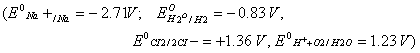2.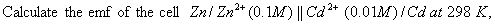(3)

Or

1. Account for the following:
1. Alkaline medium inhibits the rusting of iron. (1)
2. iron does not rust even if the zinc coating is broken in a galvanized iron pipe. (1)
2.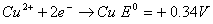1. Construct a galvanic cell using the above data.
2. For what concentration of Ag + ions will the emf of the cell be zero at 25°C, if the concentration of Cu2+ is 0.01 M? (3)
[log 3.919 = 0.593]

Q. 26. Give reasons for the following:

1. Molten aluminium bromide is poor conductor of electricity. (1)
2. Nitric oxide becomes brown when released in air. (1)
3. PCl5 is ionic in nature in the solid state. (1)
4. Ammonia acts as a ligand. (1)
5. Sulphur disappears when boiled with an aqueous solution of sodium sulphite. (1)

Or

Name the principal ore of tin or lead. Describe the different steps (along with equations for the reactions) involved in the extraction of the metal from the ore named. Name an important alloy of each, tin and lead. (21/2, 21/2)

Q. 27.

1. State two main differences between globular proteins and fibrous proteins. (2)
2. Based on their chemical composition, state how are lipids classified? Give one example of each class. (3)

Or

1. ‘Hormones are chemical messengers.’ Explain? (2)
2. Name the main disease caused due to lack of the vitamin and its source in each of the following: A, B 6 and E. (3)

 Chemistry 2006 Question Papers Class XII Delhi Outside Delhi Compartment Delhi Compartment Outside DelhiSet ISet ISet ISet I

 CBSE 2006 Question Papers Class XIIEnglishSociologyFunctional EnglishPsychologyMathematicsPhilosopyPhysicsComputer ScienceChemistryEntrepreneurshipBiologyInformatics PracticesGeographyMultimedia & Web TechnologyEconomicsBiotechnologyBusiness StudiesPhysical EducationAccountancyFine ArtsPolitical ScienceHistoryAgriculture